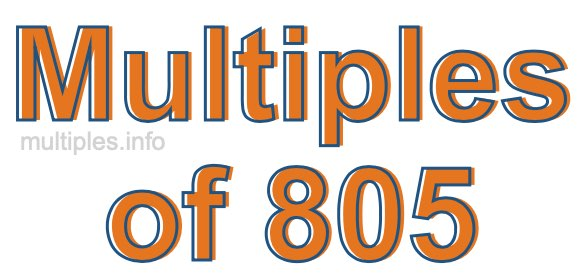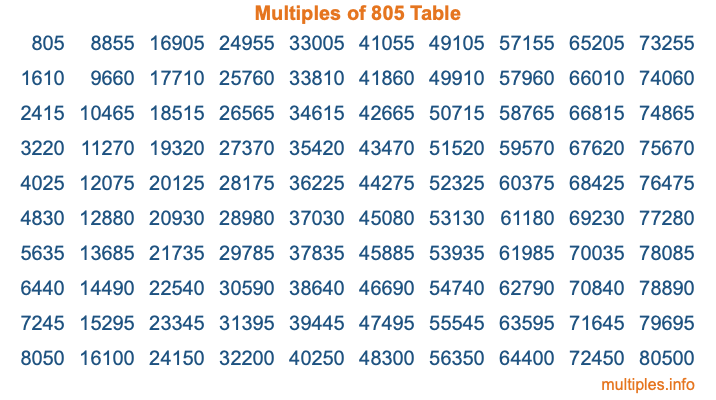Multiples of 805Welcome to the Multiples of 805 page. Here we will first teach you everything you will ever need to know about the multiples of 805, and then give you a study guide summary of everything we taught you to make sure you remember it all. Use this page to look up facts and learn information about the multiples of 805. This page will make you a multiples of eight hundred five expert!

Definition of Multiples of 805
Multiples of 805 are all the numbers that when divided by 805 equal an integer. Each of the multiples of 805 are called a multiple. A multiple of 805 is created by multiplying 805 by an integer.

Therefore, to create a list of multiples of 805, you start with 1 multiplied by 805, then 2 multiplied by 805, then 3 multiplied by 805, and so on for as long as you want. Thus, the list of the first five multiples of 805 is 805, 1610, 2415, 3220, and 4025. To see a larger list of multiples of 805, see the printable image of Multiples of 805 further down on this page. We also have a category where you can choose any nth multiple of 805.

Multiples of 805 Checker
The Multiples of 805 Checker below checks to see if any number of your choice is a multiple of 805. In other words, it checks to see if there is any number (integer) that when multiplied by 805 will equal your number. To do that, we divide your number by 805. If the the quotient is an integer, then your number is a multiple of 805.

Is  a multiple of 805?

Least Common Multiple of 805 and ...
A Least Common Multiple (LCM) is the lowest multiple that two or more numbers have in common. This is also called the smallest common multiple or lowest common multiple and is useful to know when you are adding our subtracting fractions. Enter one or more numbers below (805 is already entered) to find the LCM.

Check out our LCM Calculator if you need more details about the Least Common Multiple or if you need the LCM for different numbers for adding and subtraction fractions.

nth Multiple of 805
As we stated above, 805 is the first multiple of 805, 1610 is the second multiple of 805, 2415 is the third multiple of 805, and so on. Enter a number below to find the nth multiple of 805.

th multiple of 805

Multiples of 805 vs Factors of 805
805 is a multiple of 805 and a factor of 805, but that is where the similarities end. All postive multiples of 805 are 805 or greater than 805. All positive factors of 805 are 805 or less than 805.

Below is the beginning list of multiples of 805 and the factors of 805 so you can compare:

Multiples of 805: 805, 1610, 2415, 3220, 4025, etc.

Factors of 805: 1, 5, 7, 23, 35, 115, 161, 805

As you can see, the multiples of 805 are all the numbers that you can divide by 805 to get a whole number. The factors of 805, on the other hand, are all the whole numbers that you can multiply by another whole number to get 805.

It's also interesting to note that if a number (x) is a factor of 805, then 805 will also be a multiple of that number (x).

Multiples of 805 vs Divisors of 805
The divisors of 805 are all the integers that 805 can be divided by evenly. Below is a list of the divisors of 805.

Divisors of 805: 1, 5, 7, 23, 35, 115, 161, 805

The interesting thing to note here is that if you take any multiple of 805 and divide it by a divisor of 805, you will see that the quotient is an integer.

Multiples of 805 Table
Below is an image of the first 100 multiples of 805 in a table. The table is in chronological order, column by column. The first column has the first ten multiples of 805, the second column has the next ten multiples of 805, and so on.The Multiples of 805 Table is also referred to as the 805 Times Table or Times Table of 805. You are welcome to print out our table for your studies.

Negative Multiples of 805
Although not often discussed or needed in math, it is worth mentioning that you can make a list of negative multiples of 805 by multiplying 805 by -1, then by -2, then by -3, and so on, to get the following list of negative multiples of 805:

-805, -1610, -2415, -3220, -4025, etc.

Multiples of 805 Summary
Below is a summary of important Multiples of 805 facts that we have discussed on this page. To retain the knowledge on this page, we recommend that you read through the summary and explain to yourself or a study partner why they hold true.

There are an infinite number of multiples of 805.

A multiple of 805 divided by 805 will equal a whole number.

805 divided by a factor of 805 equals a divisor of 805.

The nth multiple of 805 is n times 805.

The largest factor of 805 is equal to the first positive multiple of 805.

805 is a multiple of every factor of 805.

805 is a multiple of 805.

A multiple of 805 divided by a divisor of 805 equals an integer.

805 divided by a divisor of 805 equals a factor of 805.

Any integer times 805 will equal a multiple of 805.

Multiples of a Number
Here you can get the multiples of another number, all with the same attention to detail as we did for multiples of 805 on this page.

Multiples of
Multiples of 806
Did you find our page about multiples of eight hundred five educational? Do you want more knowledge? Check out the multiples of the next number on our list!